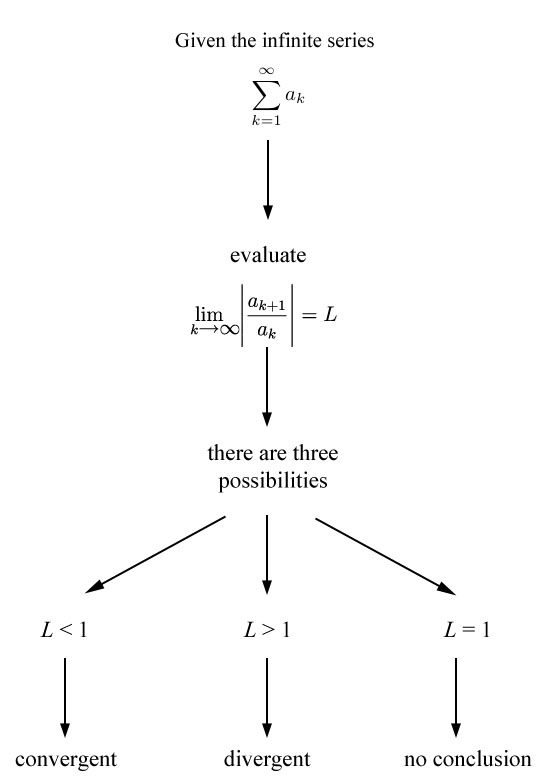# Science:Infinite Series Module/Units/Unit 2/2.4 The Ratio Test/2.4.03 A Ratio Test Flowchart

The steps involved in applying the ratio test to an infinite series are given in the flowchart below.This diagram shows the steps involved in conducting the ratio test. Observe that in the case that the limit equals one, the test does not tell us if the series is convergent or divergent.